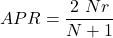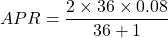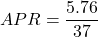## Find APR (rounded to the nearest tenth of a percent) for the loan. Purchase a living room set for $3,600 at 8% add-on interest for 3 years. Question Find APR (rounded to the nearest tenth of a percent) for the loan. Purchase a living room set for$3,600 at 8% add-on interest for 3 years.

in progress 0
6 months 2021-07-28T05:16:13+00:00 1 Answers 13 views 0

The APR for the loan 15.6%

Step-by-step explanation:

From the given information:

The annual percentage can be calculated by using the formula:where;

N = number of payments = 3 years = 12 × 3 = 36 months

r = add-on interest rate = 8% = 0.08

Replacing our value to calculate the APR, we get:APR = 0.155675

APR ≅ 15.6%